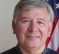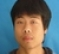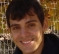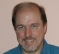## Inverse Fourier transform and discrete time fourier seriesStarted by 6 years ago8 replieslatest reply 6 years ago602 views

I have a question regarding FFT and representing a discrete periodic signal using DTFS.

From the equation of DTFS, it appears that it is same as inverse Fourier transform.

DTFS - x[n] = summation (ak*exp(j*k*w0*n)) for k = 0 to N-1 where N is the period of x[n] - eq1

iFFT - x[n] = (1/sqrt(N)*summation(X[k]*exp((j*2*pi*n*k)/N)) - eq2

Additionally, I observe that, in order to represent a discrete periodic signal using complex exponentials, one has to first find ak in eq1. To find ak, one has to first compute the FFT of x[n].

Is my observation correct?

Thanks a lot

[ - ]I have a little trouble following the question so I will offer some things that *may* help:

- A "discrete periodic signal" by virtue of being periodic will have a discrete Fourier Transform that is also periodic because it starts out discrete.  Some may argue that it's finite as opposed to periodic but I don't see an important distinction.  I find it easier to deal with and understand being periodic.

- A discrete Fourier Transform will have a periodic inverse transform.

What is the difference between a Fourier Transform of a discrete periodic sequence and a Fourier Series? It should be easy to show they are the same.  The integral becomes a summation of distinct values

I will assume the classical notation:

x[n] is a time series .. discrete by definition

X[k] is a frequency series .. discrete by definition

To me x[n] are used to compute the X[k] and X[k] are used to compute the x[n].  And, what came first, the chicken or the egg doesn't matter.

So, yes, an IFFT will result in the x[n].

But saying DTFS yields x[n] confuses me as I think of the Fourier Series computation as computing the X[k] and NOT the x[n].

Fred

[ - ]Dear Fred and others,

I think my incorrect terminology might have created confusion. I am sorry about that. So, let me re-phrase my question without using fancy/formal terms.

I assume that a discrete periodic signal can be represented using weighted sum of sinusoids. If this is true then this way of representing looks like just an inverse discrete Fourier transform.

Let me know if my understanding is correct. Further, if this is true then in order to represent the discrete periodic signal one first needs to know the weights, and in order to find weights, one has to first do a forward DFT.

Let me know if I am going in the right direction with my understanding ...

[ - ]As I tried to point out, there should be no difference when the discrete and periodic nature is accounted for.  So maybe saying a "weighted sum of sinusoids" is misleading when it should probably be " a weighted sum of sampled sinusoids".

[ - ]You've noticed they are the same expression, so $$a_k=X_k/\sqrt N$$.  Forward and inverse is a matter of sign choice.  You can choose forward with a + sign in the exponent so long as the inverse has a minus sign.  Most conventions are with - in the exponent for forward and + in the exponent for inverse.  Now that I've got you even more confused, keep working on it!!

Mike

[ - ]Hi,Sharan123,

I think FFT(fast fourier transform) is an algorithm for implementing DFT(Discrete Fourier Transform).

And IDFT - x(k)=1/N*summation (X(i）*exp(j*w0*i*K) for k=0 to N-1 where N is the period of x(n)

In my point of view, IDFT is just the DTFS, because the sequence has already expressed as the summation of periodic complex series,exp(j*k*w0),exp(j*k*2*w0)...

And yes, you need to map the periodic sequence into frequency domain to get a(K) and the method for this is DFT.

Hope this helps.

[ - ]The Discrete Fourier Transform (DFT) term is an overloaded term, as it can be a transform applied to periodic sampled signals, or a transform applied to time limited sampled signal. According to this wikipedia article

https://en.wikipedia.org/wiki/Discrete_Fourier_ser...

"...The term discrete Fourier series (DFS) is intended for use instead of DFT when the original function is periodic, defined over an infinite interval."

So its an term used to make clear that the orignal sampled signal was a periodic function.

[ - ]Normally the $$a_k$$ in your eq1 should be a time series, that comes from the problem you're trying to solve.
And as has been noted, you're kind of mixing terms, here.  The form of the discrete-time Fourier series and its inverse are the same, except for the sign in the exponent -- $$e^{j \omega t}$$ vs. $$e^{-j \omega t}$$.  Everyone defines their scaling parameters and (I guess!) the sign of the exponent term their own way, so if you are mixing algorithms from different math packages, or if you're using symbolic computations to predict what an algorithm will do, you need to make sure you're getting your scalings and signs right.